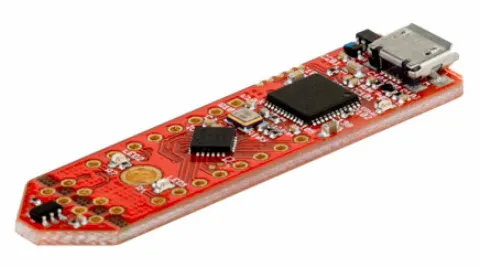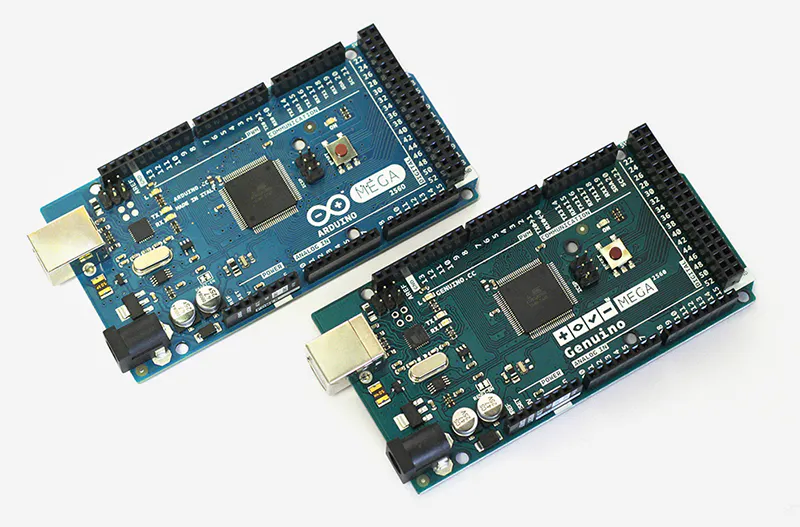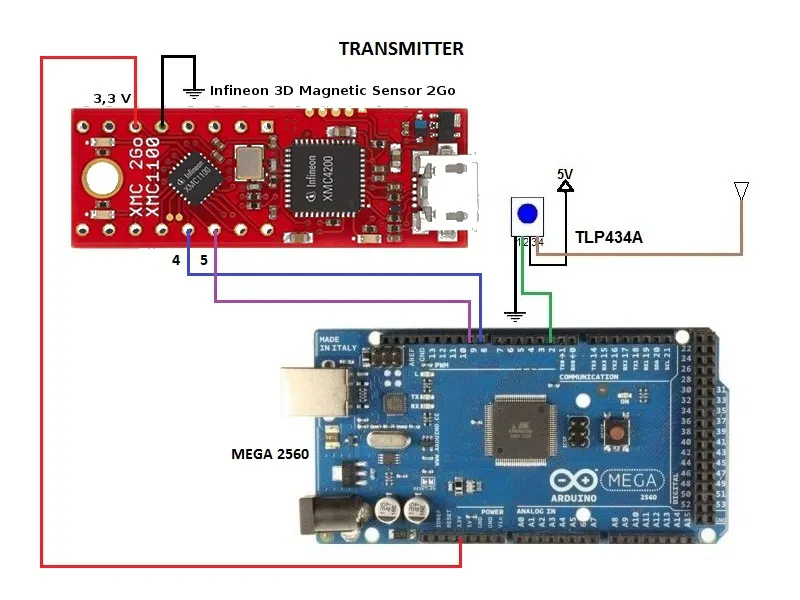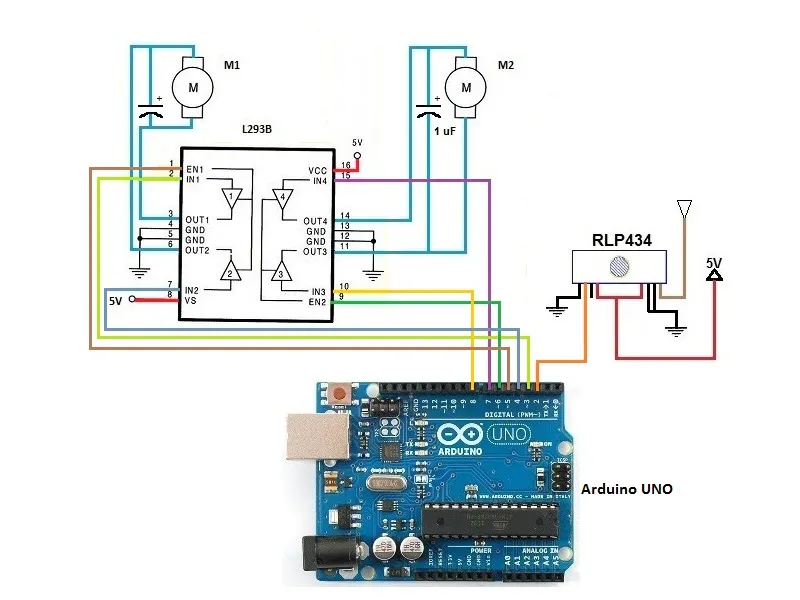# RF Remote Control With Infineon 3D Magnetic Sensor 2Go

This project shows us how we control a "Micro Robot Car" and using a RF Remote Control with "Infineon 3D Magnetic Sensor 2Go".

## Things used in this project

### Hardware componentsInfineon 3D Magnetic Sensor 2Go
×1Arduino UNO & Genuino UNO
×1Arduino Mega 2560 & Genuino Mega 2560
×1
×1
 TLP 434A RF Transmitter
×1
 L293B driver
×1

### Software apps and online servicesArduino IDE

## Schematics

### RF Transmitter## Code

### Infineon.ino

Arduino
```#include <Tle493d_w2b6.h>

Tle493d_w2b6 Tle493dMagnetic3DSensor = Tle493d_w2b6();
void setup() {
Serial.begin(9600);
pinMode (4,OUTPUT);
pinMode (5,OUTPUT);
while (!Serial);
Tle493dMagnetic3DSensor.begin();
Tle493dMagnetic3DSensor.enableTemp();
}

void loop() {
Tle493dMagnetic3DSensor.updateData();
float angle = Tle493dMagnetic3DSensor.getAzimuth() * 57.3;
int angle1 = angle;
float Norm = Tle493dMagnetic3DSensor.getNorm();

if ((angle1>=165 && angle1<=180 && Norm>=90)||(angle1>=-180 && angle1<=-165 && Norm>=90)){    // STOP
Serial.print(angle1);
Serial.println(" = STOP");
digitalWrite (4,LOW);
digitalWrite (5,LOW);
delay (10);
}

else if (angle1>=-105 && angle1<=-75 && Norm>=90){    // RIGHT
Serial.print(angle1);
Serial.println(" = RIGHT");
digitalWrite (4,LOW);
digitalWrite (5,HIGH);
delay (10);
}

if (angle1>=-15 && angle1<=15 && Norm>=90){    // FORWARD
Serial.print(angle1);
Serial.println(" = FORWARD");
digitalWrite (4,HIGH);
digitalWrite (5,LOW);
delay(10);
}

else if (angle1>=75 && angle1<=105 && Norm>=90){    // LEFT
Serial.print(angle1);
Serial.println(" = LEFT");
digitalWrite (4,HIGH);
digitalWrite (5,HIGH);
delay (10);
}
}
```

### Tx_mega.ino

Arduino
```#include <VirtualWire.h>

#define SWITCH1 8   //input for SWITCH1
#define SWITCH2 9   //input for SWITCH2

void setup()
{
Serial.begin(9600);
Serial.println("Tx RF");
pinMode (SWITCH1,INPUT);
pinMode (SWITCH2,INPUT);

// Se inicializa el RF
vw_setup(2000); // bps
vw_set_tx_pin(2); //output RF
}

void loop()
{
char dato;

dato = 'S';
vw_send((uint8_t*)dato,sizeof(dato));
vw_wait_tx();
Serial.println("STOP");
}

dato = 'R';
vw_send((uint8_t*)dato,sizeof(dato));
vw_wait_tx();
Serial.println("RIGHT");
}

dato = 'F';
vw_send((uint8_t*)dato,sizeof(dato));
vw_wait_tx();
Serial.println("FORWARD");
}

dato = 'L';
vw_send((uint8_t*)dato,sizeof(dato));
vw_wait_tx();
Serial.println("LEFT");
}
delay(200);
}
```

### Rx_arduino.ino

Arduino
```#include <VirtualWire.h>

void setup()
{
Serial.begin(9600);  // Debugging only
Serial.println("setup");

// Se inicializa el RF
vw_setup(2000);  // bps
vw_set_rx_pin(2);  //Pin 2 as input RF
vw_rx_start();       // start

pinMode(3,OUTPUT);
pinMode(4,OUTPUT);
pinMode(5,OUTPUT);
pinMode(6,OUTPUT);
pinMode(7,OUTPUT);
pinMode(8,OUTPUT);
}

void loop()
{
uint8_t dato;
uint8_t datoleng=1;
//verufy RF data
if (vw_get_message(&dato,&datoleng))
{
if((char)dato=='S')   // STOP
{
digitalWrite(3,LOW);
digitalWrite(4,LOW);
digitalWrite(5,LOW);  //en1
digitalWrite(6,LOW);  //en2
digitalWrite(7,LOW);
digitalWrite(8,LOW);
delay(10);
Serial.println("STOP");
}

if((char)dato=='R')   // RIGHT
{
digitalWrite(3,HIGH);
digitalWrite(4,LOW);
digitalWrite(5,HIGH);  //en1
digitalWrite(6,HIGH);  //en2
digitalWrite(7,HIGH);
digitalWrite(8,LOW);
delay(10);
Serial.println("RIGHT");
}

if((char)dato=='L')   // LEFT
{
digitalWrite(3,LOW);
digitalWrite(4,HIGH);
digitalWrite(5,HIGH);  //en1
digitalWrite(6,HIGH);  //en2
digitalWrite(7,LOW);
digitalWrite(8,HIGH);
delay(10);
Serial.println("LEFT");
}

if((char)dato=='F')   // FORWARD
{
digitalWrite(3,HIGH);
digitalWrite(4,LOW);
digitalWrite(5,HIGH);  //en1
digitalWrite(6,HIGH);  //en2
digitalWrite(7,LOW);
digitalWrite(8,HIGH);
delay(10);
Serial.println("FORWARD");
}
}
}
```

## Credits

### Guillermo Alberto Perez Guillen

27 projects • 27 followers
Communications and Electronics Engineering, and Writer... "Don´t Expect Different Results If Your Habits Are The Same" A Einstein